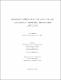## Strings on complex multiplication tori and rational conformal field theory with matrix level2013
##### Authors
Nassar, Ali
University of Lethbridge. Faculty of Arts and Science
##### Publisher
Lethbridge, Alta. : University of Lethbridge, Dept. of Physics and Astronomy
##### Abstract
Conformal invariance in two dimensions is a powerful symmetry. Two-dimensional quantum field theories which enjoy conformal invariance, i.e., conformal field theories (CFTs) are of great interest in both physics and mathematics. CFTs describe the dynamics of the world sheet in string theory where conformal symmetry arises as a remnant of reparametrization invariance of the world-sheet coordinates. In statistical mechanics, CFTs describe the critical points of second order phase transitions. On the mathematics side, conformal symmetry gives rise to infinite dimensional chiral algebras like the Virasoro algebra or extensions thereof. This gave rise to the study of vertex operator algebras (VOAs) which is an interesting branch of mathematics. Rational conformal theories are a simple class of CFTs characterized by a finite number of representations of an underlying chiral algebra. The chiral algebra leads to a set of Ward identities which gives a complete non-perturbative solution of the RCFT. Identifying the chiral algebra of an RCFT is a very important step in solving it. Particularly interesting RCFTs are the ones which arise from the compactificatin of string theory as -models on a target manifold M. At generic values of the geo- metric moduli of M, the corresponding CFT is not rational. Rationality can arise at particular values of the modui of M. At these special values of the moduli, the chiral algebra is extended. This interplay between the geometric picture and the algebraic description encoded in the chiral algebra makes CFTs/RCFTs a perfect link between physics and mathematics. It is always useful to find a geometric interpretation of a chiral algebra in terms of a -model on some target manifold M. Then the next step is to figure out the conditions on the geometric moduli of M which gives a RCFT. In this thesis, we limit ourselves to the simplest class of string compactifications, i.e., strings on tori. As Gukov and Vafa proved, rationality selects the complex- multiplication tori. On the other hand, the study of the matrix-level affine algebra Um;K is motivated by conformal field theory and the fractional quantum Hall effect. Gannon completed the classification of Um;K modular-invariant partition functions. Here we connect the algebra U2;K to strings on 2-tori describable by rational conformal field theories. We point out that the rational conformal field theories describing strings on complex- multiplication tori have characters and partition functions identical to those of the matrix-level algebra Um;K. This connection makes obvious that the rational theories are dense in the moduli space of strings on Tm, and may prove useful in other ways.
##### Keywords
rational conformal field theory , symmetry principles , complex multiplication tori , string theory , Conformal invariants , String models , Quantum field theory , Torus (Geometry) , Mathematical physics , Mathematical analysis , General relativity (Physics) , Dissertations, Academic